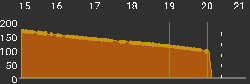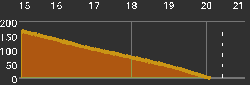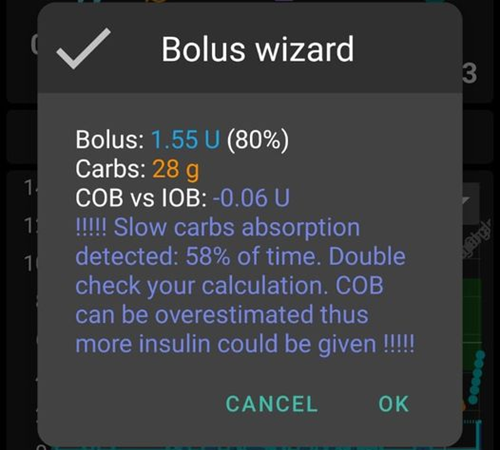# COB calculation

## How does AAPS calculate the COB value?

When carbs are entered as part of a meal or correction, AAPS adds them to the current carbs on board (COB). AAPS then absorbs (removes) carbs based on observed deviations to BG values. The rate of absorption depends on the carb sensitivity factor. This is not a profile value but is calculated as ISF/IC and is how many mg/dl 1g of carbs will raise your BG.

The formula is: `absorbed_carbs = deviation * ic / isf` It means:

• increasing ic will increase carbs absorbed every 5 minutes thus shorten total time of absorption

• increasing isf will decrease carbs absorbed every 5 minutes thus prolong total time of absorption

• changing profile % increase/decrease both values thus has no impact on carbs absorption time

For example, if your profile ISF is 100 and your IC is 5, your CSF would be 20. For every 20 mg/dl your BG goes up, 1g of carbs are absorbed by AAPS. Positive IOB also effects this calculation. So, if AAPS expected your BG to go down by 20 mg/dl because of IOB and it instead stayed flat, it would also absorb 1g of carbs.

Carbs will also be absorbed via the methods described below based on what sensitivity algorithm is used.

### Oref1

Unabsorbed carbs are cut off after specified time### AAPS, WeightedAverage

absorption is calculated to have `COB == 0` after specified timeIf minimal carbs absorption (min_5m_carbimpact) is used instead of value calculated from BG deviations, an orange dot appears on COB graph.

## Detection of wrong COB values

AAPS warns you if you are about to bolus with COB from a previous meal and the algorithm thinks that current COB calculation could be wrong. In this case it will give you an additional hint on the confirmation screen after usage of bolus wizard.

### How does AAPS detect wrong COB values?

Normally AAPS detects carb absorption through BG deviations. In case you entered carbs but AAPS cannot see their estimated absorption through BG deviations, it will use the min_5m_carbimpact method to calculate the absorption instead (so called ‘fallback’). As this method calculates only the minimal carb absorption without considering BG deviations, it might lead to incorrect COB values.In the screenshot above, 41% of time the carb absorption was mathematically calculated by the min_5m_carbimpact instead of the value detected from deviations. This means that maybe you are having less carbs on board than calculated by the algorithm.

### How to deal with this warning?

• Consider to cancel the treatment - press Cancel instead of OK.

• Calculate your upcoming meal again with bolus wizard leaving COB unticked.

• In case you are sure you need a correction bolus, enter it manually.

• In any case be careful not to overdose!

### Why does the algorithm not detect COB correctly?

• Maybe you overestimated carbs when entering them.

• Activity / exercise after your previous meal